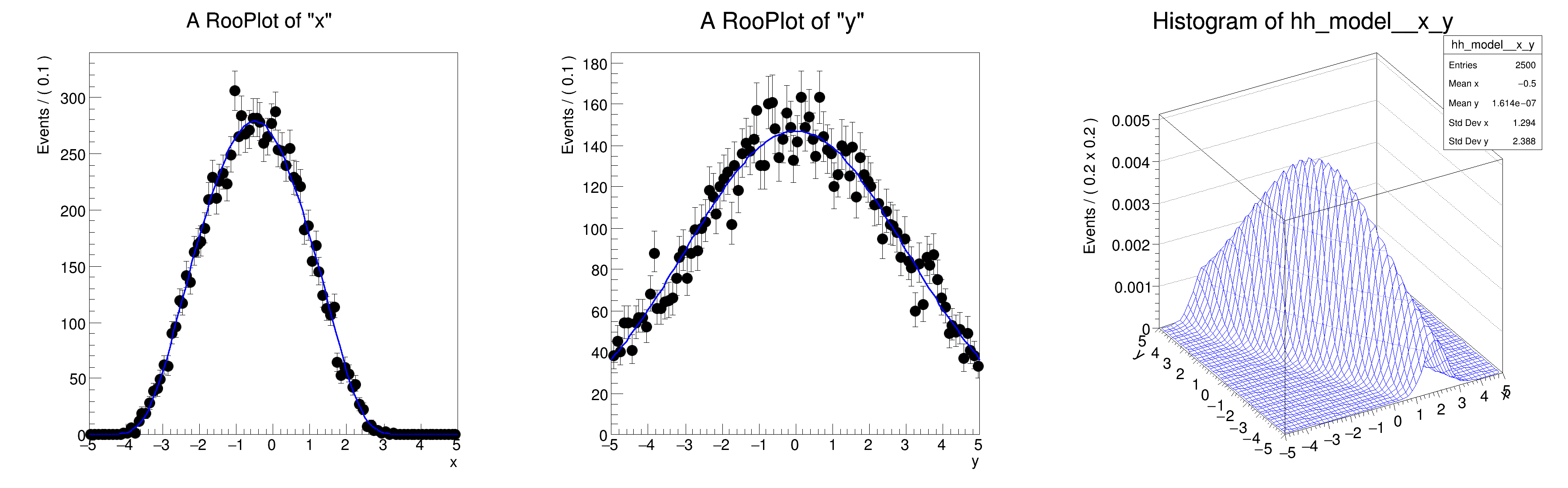ROOT   Reference Guiderf305_condcorrprod.C File Reference

## Detailed Description

Multidimensional models: multi-dimensional p.d.f.s with conditional p.d.fs in product

pdf = gauss(x,f(y),sx | y ) * gauss(y,ms,sx) with f(y) = a0 + a1*y␛[1mRooFit v3.60 -- Developed by Wouter Verkerke and David Kirkby␛[0m
Copyright (C) 2000-2013 NIKHEF, University of California & Stanford University
[#1] INFO:Plotting -- RooAbsReal::plotOn(model) plot on x integrates over variables (y)
[#1] INFO:NumericIntegration -- RooRealIntegral::init([gaussy_NORM[y]_X_gaussx_NORM[x]]_Int[y]) using numeric integrator RooIntegrator1D to calculate Int(y)
[#1] INFO:Plotting -- RooAbsReal::plotOn(model) plot on y integrates over variables (x)
#include "RooRealVar.h"
#include "RooDataSet.h"
#include "RooGaussian.h"
#include "RooConstVar.h"
#include "RooPolyVar.h"
#include "RooProdPdf.h"
#include "RooPlot.h"
#include "TCanvas.h"
#include "TAxis.h"
#include "TH1.h"
using namespace RooFit;
{
// C r e a t e c o n d i t i o n a l p d f g x ( x | y )
// -----------------------------------------------------------
// Create observables
RooRealVar x("x", "x", -5, 5);
RooRealVar y("y", "y", -5, 5);
// Create function f(y) = a0 + a1*y
RooRealVar a0("a0", "a0", -0.5, -5, 5);
RooRealVar a1("a1", "a1", -0.5, -1, 1);
RooPolyVar fy("fy", "fy", y, RooArgSet(a0, a1));
// Create gaussx(x,f(y),sx)
RooRealVar sigmax("sigma", "width of gaussian", 0.5);
RooGaussian gaussx("gaussx", "Gaussian in x with shifting mean in y", x, fy, sigmax);
// C r e a t e p d f g y ( y )
// -----------------------------------------------------------
// Create gaussy(y,0,5)
RooGaussian gaussy("gaussy", "Gaussian in y", y, RooConst(0), RooConst(3));
// C r e a t e p r o d u c t g x ( x | y ) * g y ( y )
// -------------------------------------------------------
// Create gaussx(x,sx|y) * gaussy(y)
RooProdPdf model("model", "gaussx(x|y)*gaussy(y)", gaussy, Conditional(gaussx, x));
// S a m p l e , f i t a n d p l o t p r o d u c t p d f
// ---------------------------------------------------------------
// Generate 1000 events in x and y from model
RooDataSet *data = model.generate(RooArgSet(x, y), 10000);
// Plot x distribution of data and projection of model on x = Int(dy) model(x,y)
RooPlot *xframe = x.frame();
data->plotOn(xframe);
model.plotOn(xframe);
// Plot x distribution of data and projection of model on y = Int(dx) model(x,y)
RooPlot *yframe = y.frame();
data->plotOn(yframe);
model.plotOn(yframe);
// Make two-dimensional plot in x vs y
TH1 *hh_model = model.createHistogram("hh_model", x, Binning(50), YVar(y, Binning(50)));
hh_model->SetLineColor(kBlue);
// Make canvas and draw RooPlots
TCanvas *c = new TCanvas("rf305_condcorrprod", "rf05_condcorrprod", 1200, 400);
c->Divide(3);
c->cd(1);
xframe->GetYaxis()->SetTitleOffset(1.6);
xframe->Draw();
c->cd(2);
yframe->GetYaxis()->SetTitleOffset(1.6);
yframe->Draw();
c->cd(3);
hh_model->GetZaxis()->SetTitleOffset(2.5);
hh_model->Draw("surf");
}
#define c(i)
Definition: RSha256.hxx:101
@ kBlue
Definition: Rtypes.h:64
virtual RooPlot * plotOn(RooPlot *frame, const RooCmdArg &arg1=RooCmdArg::none(), const RooCmdArg &arg2=RooCmdArg::none(), const RooCmdArg &arg3=RooCmdArg::none(), const RooCmdArg &arg4=RooCmdArg::none(), const RooCmdArg &arg5=RooCmdArg::none(), const RooCmdArg &arg6=RooCmdArg::none(), const RooCmdArg &arg7=RooCmdArg::none(), const RooCmdArg &arg8=RooCmdArg::none()) const
Calls RooPlot* plotOn(RooPlot* frame, const RooLinkedList& cmdList) const ;.
Definition: RooAbsData.cxx:546
RooArgSet is a container object that can hold multiple RooAbsArg objects.
Definition: RooArgSet.h:28
RooDataSet is a container class to hold unbinned data.
Definition: RooDataSet.h:33
Plain Gaussian p.d.f.
Definition: RooGaussian.h:25
A RooPlot is a plot frame and a container for graphics objects within that frame.
Definition: RooPlot.h:44
TAxis * GetYaxis() const
Definition: RooPlot.cxx:1277
virtual void Draw(Option_t *options=0)
Draw this plot and all of the elements it contains.
Definition: RooPlot.cxx:712
Class RooPolyVar is a RooAbsReal implementing a polynomial in terms of a list of RooAbsReal coefficie...
Definition: RooPolyVar.h:28
RooProdPdf is an efficient implementation of a product of PDFs of the form.
Definition: RooProdPdf.h:31
RooRealVar represents a variable that can be changed from the outside.
Definition: RooRealVar.h:35
virtual void SetTitleOffset(Float_t offset=1)
Set distance between the axis and the axis title.
Definition: TAttAxis.cxx:294
virtual void SetLineColor(Color_t lcolor)
Set the line color.
Definition: TAttLine.h:40
The Canvas class.
Definition: TCanvas.h:27
The TH1 histogram class.
Definition: TH1.h:56
TAxis * GetZaxis()
Definition: TH1.h:318
virtual void Draw(Option_t *option="")
Draw this histogram with options.
Definition: TH1.cxx:2998
RooCmdArg YVar(const RooAbsRealLValue &var, const RooCmdArg &arg=RooCmdArg::none())
RooConstVar & RooConst(Double_t val)
RooCmdArg Conditional(const RooArgSet &pdfSet, const RooArgSet &depSet, Bool_t depsAreCond=kFALSE)
RooCmdArg Binning(const RooAbsBinning &binning)
Double_t y[n]
Definition: legend1.C:17
Double_t x[n]
Definition: legend1.C:17
The namespace RooFit contains mostly switches that change the behaviour of functions of PDFs (or othe...
Date
07/2008

Definition in file rf305_condcorrprod.C.